# simply supported beam with point loads

In this tutorial we will:

• calculate shearing forces (V) and bending moments (M) for a simply supported beam with point loads
• demonstrate the general relationship between V and M
• construct shearing force and bending moment diagrams.

Consider this example of a simply supported beam with three point loads.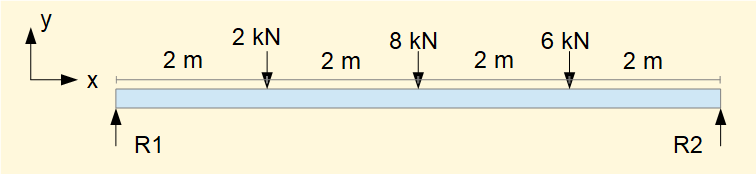Reactions R1 and R2 are calculated as follows (upward forces +ve}.

Σ vertical forces = 0

Gives    R1 + R2 - 2 - 8 - 6 = 0        Thus R1 + R2 = 16 kN    --------- (i)

Σ of moments about left hand end = 0

Clockwise moments are +ve

Gives    (2 x 2) + (8 x 4) + (6 x 6) - (R2 x 8) = 0

Thus    (8 x R2) = 4 +32 +36 = 72        Gives    R2 = 9 kN

Substitute R2 in (i)        Gives    R1 = 7 kN

We will now calculate shearing forces V and bending moments M along the beam using free body diagrams from datum x = 0 .

Firstly note that  M = 0 at  x = 0 and at x = 8 since points of simple support cannot sustain bending moments.

Now consider free body diagrams for sections of the beam between each point load, say at x=1, x=3. x=5, x=7 and take Σ vertical forces = 0 and Σ moments = 0 for each section.

Firstly consider the section from x = 0 to x = 1.  Assume that we do not know the signs of V and M.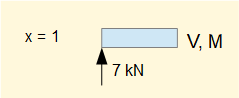Σ vertical forces = 0

±V + 7 = 0 Thus V (appears to be) = -7 kN

However, the sign convention for shearing forces on an element of the beam is: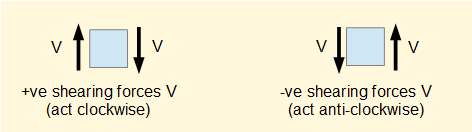We must reverse the sign of V so in this case V is +ve    thus    V = + 7 kN

Σ moments = 0  (at position x = 1)

R1 x 1) ± M = 0

Gives    ( 7 x 1) ± M = 0     Thus M (appears to be) = -7 kNm

However, the sign convention for +ve and -ve bending moments on an element of the beam is: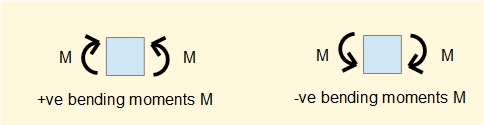We must reverse the sign of M So in this case    M is +ve    thus    M = +7 kNm

The free body diagram is as follows showing +ve bending and +ve shear.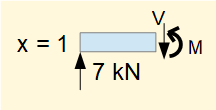Note that the conflict regarding sign conventions can be avoided if the direction of the x axis is reversed and the datum point for taking sections is from the right hand end of the beam. On balance I prefer using the conventional direction for the x axis.

Sections from x = 0 to x = 3, x = 5 and x = 7 follow below.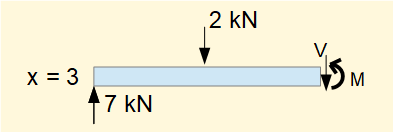At x = 3     V = 5 kN    M = 19 kNm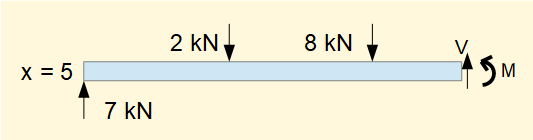At x = 5     V = -3 kN    M = 21 kNm     (note V changes sign from + to - )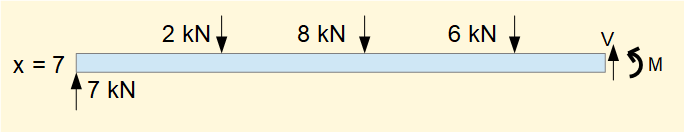At x = 7     V = -9 kN    M = 9kNm

Note that V is constant between the point loads, i.e.

for 0 < x < 2    V = 7 kN
for 2 < x < 4    V = 5 kN
for 4 < x < 6    V = -3 kN
for 6 < x < 8    V = -9 kN

At positions of point loads and reaction forces V is indeterminate, i.e. there is a step change.

We plot values for V on a shearing force diagram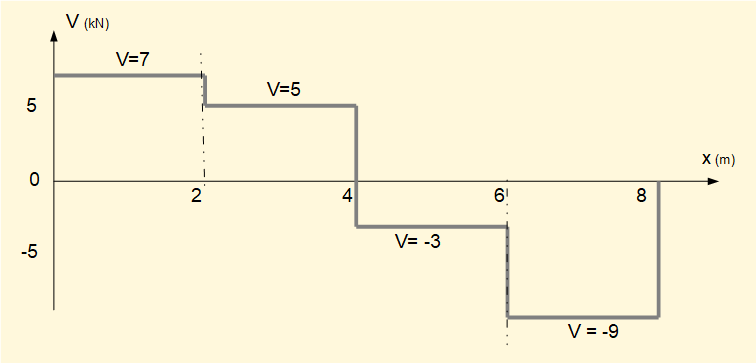Before we construct the equivalent bending moment diagram consider the changes in M and values of V for sections along the beam.

By calculation:
at x = 2    M = 14 kNm
at x = 4    M = 24 kNm
at x = 6    M = 18 kNm

 Section of beam ( Δx = 1 m) ΔM (kNm) ΔM/Δx V (kN) x = 0 to 1 +7 +7 +7 x = 1 to 2 +7 +7 +7 x = 2 to 3 +5 +5 +5 x = 3 to 4 +5 +5 +5 x = 4 to 5 -3 -3 -3 x = 5 to 6 -3 -3 -3 x = 6 to 7 -9 -9 -9 x = 7 to 8 -9 -9 -9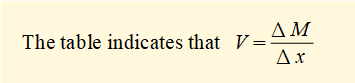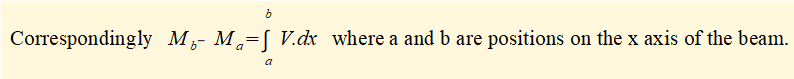We can now construct the bending moment diagram for the beam in this example noting that the slope of M is constant between the point loads and equals V (as shown in the above table).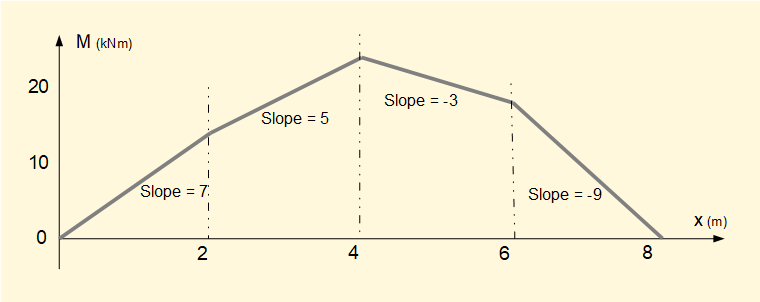I welcome feedback at:

### Tutorials - mechanical vibrations

###### Forced vibrations with damping

Alistair's tutorials 2019